# Timeseries Classification: KNN & DTW¶

Mark Regan

When it comes to building a classification algorithm, analysts have a broad range of open source options to choose from. However, for time series classification, there are less out-of-the box solutions. Many of the typical classification algorithms (Logistic Regression, Random Forests, SVM etc) are applicable to time-series data when the temporal data structure is abstracted away through feature engineering.

My experience with this approach has been poor. The full timeseries feature vector contains a large amount of valuable information that can be lost through the process of flattening the datastructure.

I began researching the domain of time series classification and was intrigued by a recommended technique called K Nearest Neighbors and Dynamic Time Warping. A meta analysis completed by Mitsa (2010) suggests that when it comes to timeseries classification, 1 Nearest Neighbor (K=1) and Dynamic Timewarping is very difficult to beat .

## K Nearest Neighbors & Dynamic Time Warping¶

In :
from IPython.display import Image
Image('images/dtw_knn_schematic.png', width=780)

Out: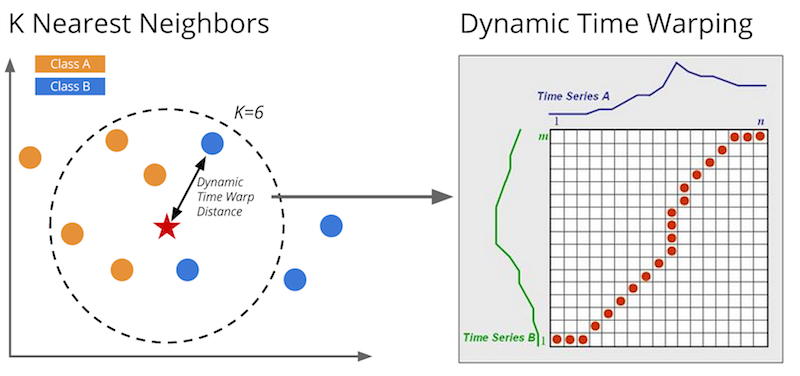K Nearest Neighbors is a classification algorithm familiar to many. It takes a unlabled observation (the star above) and compares it to a population of labled observations (blue and orange circles). By finding the K nearest circles to the star, we can infer the class label for the star through majority voting.

We can also use KNN in the context of time series data. The question becomes, "how do we compute the distance between two timeseries sequences"? "Dynamic Time Warping" is a technique that was heavily used for speech recognition in the 80s. The DTW algorithm finds the optimum alignment between two sequences of observations by warping the time dimension with certain constraints.

Because of this temporal dimension warping, DTW is good for classifying sequences that have different frequences or that are out of phase. Later we'll see how we can use DTW to classify whether a person is walking, lying down, sitting etc.

The above right diagram shows how a DTW distance matrix representation. Each cell is computed by measuring the distance between $A_i$ and $B_j$. The red path represents the shortest path and hence optimum alignment of the two sequences.

$$DTW_{AB} = SUM(shortest\ paths_{AB})$$

#### Max Warping Window Allowed¶

Computing the full distance matrix between A and B scales with $O(n^2)$, where n is the number of sequences in A and B. This performance can be improved by constraining the amount of warping allowed. This limits the number of cells that need to be computed in the DTW distance matrix. Research by Keogh et al has shown that a warping window not only improves performance but also improves classification accuracy .

In :
Image('images/max_window_warping.png')

Out: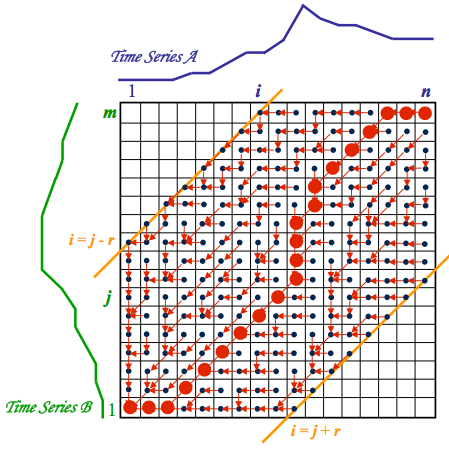#### Coded in Python¶

The below python code represents the KNN & DTW classification algorithm. The following key methods are described:

Method Description
_dtw_distance computes the Dynamic Time Warping distance between two sequences
_dist_matrix computes the distance matrix between $A$ and $B$
predict uses both of the above methods to compute the class labels and probability of dataset $B$ through K Nearest Neighbors
In :
import sys
import collections
import itertools
import numpy as np
import matplotlib.pyplot as plt
from scipy.stats import mode
from scipy.spatial.distance import squareform

plt.style.use('bmh')
%matplotlib inline

try:
from IPython.display import clear_output
have_ipython = True
except ImportError:
have_ipython = False

class KnnDtw(object):
"""K-nearest neighbor classifier using dynamic time warping
as the distance measure between pairs of time series arrays

Arguments
---------
n_neighbors : int, optional (default = 5)
Number of neighbors to use by default for KNN

max_warping_window : int, optional (default = infinity)
Maximum warping window allowed by the DTW dynamic
programming function

subsample_step : int, optional (default = 1)
Step size for the timeseries array. By setting subsample_step = 2,
the timeseries length will be reduced by 50% because every second
item is skipped. Implemented by x[:, ::subsample_step]
"""

def __init__(self, n_neighbors=5, max_warping_window=10000, subsample_step=1):
self.n_neighbors = n_neighbors
self.max_warping_window = max_warping_window
self.subsample_step = subsample_step

def fit(self, x, l):
"""Fit the model using x as training data and l as class labels

Arguments
---------
x : array of shape [n_samples, n_timepoints]
Training data set for input into KNN classifer

l : array of shape [n_samples]
Training labels for input into KNN classifier
"""

self.x = x
self.l = l

def _dtw_distance(self, ts_a, ts_b, d = lambda x,y: abs(x-y)):
"""Returns the DTW similarity distance between two 2-D
timeseries numpy arrays.

Arguments
---------
ts_a, ts_b : array of shape [n_samples, n_timepoints]
Two arrays containing n_samples of timeseries data
whose DTW distance between each sample of A and B
will be compared

d : DistanceMetric object (default = abs(x-y))
the distance measure used for A_i - B_j in the
DTW dynamic programming function

Returns
-------
DTW distance between A and B
"""

# Create cost matrix via broadcasting with large int
ts_a, ts_b = np.array(ts_a), np.array(ts_b)
M, N = len(ts_a), len(ts_b)
cost = sys.maxint * np.ones((M, N))

# Initialize the first row and column
cost[0, 0] = d(ts_a, ts_b)
for i in xrange(1, M):
cost[i, 0] = cost[i-1, 0] + d(ts_a[i], ts_b)

for j in xrange(1, N):
cost[0, j] = cost[0, j-1] + d(ts_a, ts_b[j])

# Populate rest of cost matrix within window
for i in xrange(1, M):
for j in xrange(max(1, i - self.max_warping_window),
min(N, i + self.max_warping_window)):
choices = cost[i - 1, j - 1], cost[i, j-1], cost[i-1, j]
cost[i, j] = min(choices) + d(ts_a[i], ts_b[j])

# Return DTW distance given window
return cost[-1, -1]

def _dist_matrix(self, x, y):
"""Computes the M x N distance matrix between the training
dataset and testing dataset (y) using the DTW distance measure

Arguments
---------
x : array of shape [n_samples, n_timepoints]

y : array of shape [n_samples, n_timepoints]

Returns
-------
Distance matrix between each item of x and y with
shape [training_n_samples, testing_n_samples]
"""

# Compute the distance matrix
dm_count = 0

# Compute condensed distance matrix (upper triangle) of pairwise dtw distances
# when x and y are the same array
if(np.array_equal(x, y)):
x_s = np.shape(x)
dm = np.zeros((x_s * (x_s - 1)) // 2, dtype=np.double)

p = ProgressBar(shape(dm))

for i in xrange(0, x_s - 1):
for j in xrange(i + 1, x_s):
dm[dm_count] = self._dtw_distance(x[i, ::self.subsample_step],
y[j, ::self.subsample_step])

dm_count += 1
p.animate(dm_count)

# Convert to squareform
dm = squareform(dm)
return dm

# Compute full distance matrix of dtw distnces between x and y
else:
x_s = np.shape(x)
y_s = np.shape(y)
dm = np.zeros((x_s, y_s))
dm_size = x_s*y_s

p = ProgressBar(dm_size)

for i in xrange(0, x_s):
for j in xrange(0, y_s):
dm[i, j] = self._dtw_distance(x[i, ::self.subsample_step],
y[j, ::self.subsample_step])
# Update progress bar
dm_count += 1
p.animate(dm_count)

return dm

def predict(self, x):
"""Predict the class labels or probability estimates for
the provided data

Arguments
---------
x : array of shape [n_samples, n_timepoints]
Array containing the testing data set to be classified

Returns
-------
2 arrays representing:
(1) the predicted class labels
(2) the knn label count probability
"""

dm = self._dist_matrix(x, self.x)

# Identify the k nearest neighbors
knn_idx = dm.argsort()[:, :self.n_neighbors]

# Identify k nearest labels
knn_labels = self.l[knn_idx]

# Model Label
mode_data = mode(knn_labels, axis=1)
mode_label = mode_data
mode_proba = mode_data/self.n_neighbors

return mode_label.ravel(), mode_proba.ravel()

class ProgressBar:
"""This progress bar was taken from PYMC
"""
def __init__(self, iterations):
self.iterations = iterations
self.prog_bar = '[]'
self.fill_char = '*'
self.width = 40
self.__update_amount(0)
if have_ipython:
self.animate = self.animate_ipython
else:
self.animate = self.animate_noipython

def animate_ipython(self, iter):
print '\r', self,
sys.stdout.flush()
self.update_iteration(iter + 1)

def update_iteration(self, elapsed_iter):
self.__update_amount((elapsed_iter / float(self.iterations)) * 100.0)
self.prog_bar += '  %d of %s complete' % (elapsed_iter, self.iterations)

def __update_amount(self, new_amount):
percent_done = int(round((new_amount / 100.0) * 100.0))
all_full = self.width - 2
num_hashes = int(round((percent_done / 100.0) * all_full))
self.prog_bar = '[' + self.fill_char * num_hashes + ' ' * (all_full - num_hashes) + ']'
pct_place = (len(self.prog_bar) // 2) - len(str(percent_done))
pct_string = '%d%%' % percent_done
self.prog_bar = self.prog_bar[0:pct_place] + \
(pct_string + self.prog_bar[pct_place + len(pct_string):])

def __str__(self):
return str(self.prog_bar)


#### Measuring the DTW distance¶

The DTW distance between two sequences can be calculated using the _dtw_distance() method

In :
time = np.linspace(0,20,1000)
amplitude_a = 5*np.sin(time)
amplitude_b = 3*np.sin(time + 1)

m = KnnDtw()
distance = m._dtw_distance(amplitude_a, amplitude_b)

fig = plt.figure(figsize=(12,4))
_ = plt.plot(time, amplitude_a, label='A')
_ = plt.plot(time, amplitude_b, label='B')
_ = plt.title('DTW distance between A and B is %.2f' % distance)
_ = plt.ylabel('Amplitude')
_ = plt.xlabel('Time')
_ = plt.legend()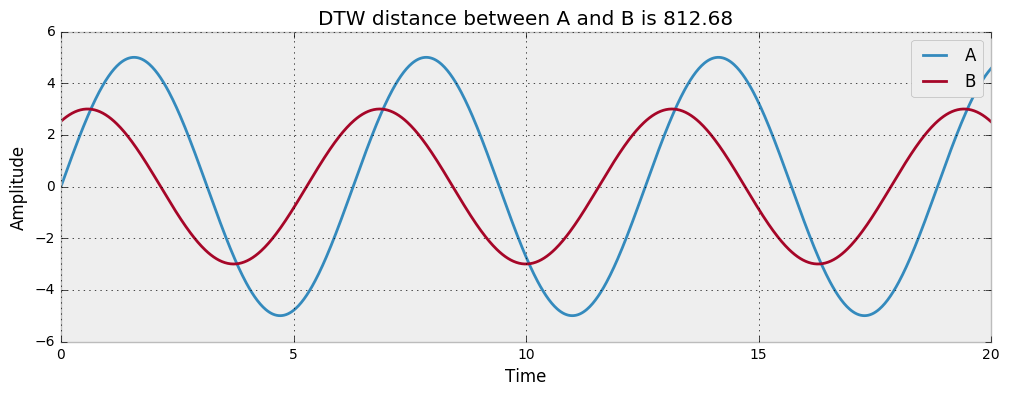It is also possible to compute the distance between each pair of two collections of inputs by using the _dist_matrix() method. These are the two methods which underpin the KnnDtw() classification algorithm. In the next section we will use the fit() and predict() method to train our classifier and predict class labels forunseen data.

In :
m._dist_matrix(np.random.random((4,50)), np.random.random((4,50)))

[****************100%******************]  16 of 16 complete
Out:
array([[  8.23266932,  10.69306752,   8.64633783,   9.32063527],
[  8.10594233,   8.28282499,   8.32599861,  10.05961871],
[  7.85953015,   8.71231086,   9.12059861,   8.74224623],
[ 10.10324773,  10.13287959,   8.98606925,  11.63278765]])

## Human Activity Recognition Dataset¶

The Human Activity Recognition Dataset (HAR) dataset is chosen to test the classification performance of DTW & KNN .

In :
Image('images/stand-sit-run.jpg')

Out: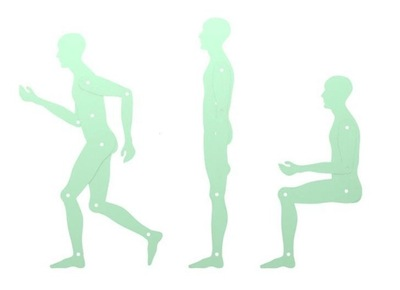The experiments were carried out with a group of 30 volunteers within an age bracket of 19-48 years. Each person performed six activities (walking, walking upstairs, walking downstairs, sitting, standing and laying) wearing a smartphone (Samsung Galaxy S II) on the waist. Using its embedded accelerometer and gyroscope, we captured 3-axial linear acceleration and 3-axial angular velocity at a constant rate of 50Hz. The experiments have been video-recorded to label the data manually.

The remainder of this analysis uses a training and test dataset provided by the authors. They have combined the above timeseries signals and created a single timeseries feature vector. Unfortunately, their methodology is not described.

#### Import the HAR dataset¶

In :
# Import the HAR dataset
x_train_file = open('data/UCI-HAR-Dataset/train/X_train.txt', 'r')
y_train_file = open('data/UCI-HAR-Dataset/train/y_train.txt', 'r')

x_test_file = open('data/UCI-HAR-Dataset/test/X_test.txt', 'r')
y_test_file = open('data/UCI-HAR-Dataset/test/y_test.txt', 'r')

# Create empty lists
x_train = []
y_train = []
x_test = []
y_test = []

# Mapping table for classes
labels = {1:'WALKING', 2:'WALKING UPSTAIRS', 3:'WALKING DOWNSTAIRS',
4:'SITTING', 5:'STANDING', 6:'LAYING'}

# Loop through datasets
for x in x_train_file:
x_train.append([float(ts) for ts in x.split()])

for y in y_train_file:
y_train.append(int(y.rstrip('\n')))

for x in x_test_file:
x_test.append([float(ts) for ts in x.split()])

for y in y_test_file:
y_test.append(int(y.rstrip('\n')))

# Convert to numpy for efficiency
x_train = np.array(x_train)
y_train = np.array(y_train)
x_test = np.array(x_test)
y_test = np.array(y_test)


#### Visualizing sample observations from the HAR dataset¶

In :
plt.figure(figsize=(11,7))
colors = ['#D62728','#2C9F2C','#FD7F23','#1F77B4','#9467BD',
'#8C564A','#7F7F7F','#1FBECF','#E377C2','#BCBD27']

for i, r in enumerate([0,27,65,100,145,172]):
plt.subplot(3,2,i+1)
plt.plot(x_train[r][:100], label=labels[y_train[r]], color=colors[i], linewidth=2)
plt.xlabel('Samples @50Hz')
plt.legend(loc='upper left')
plt.tight_layout()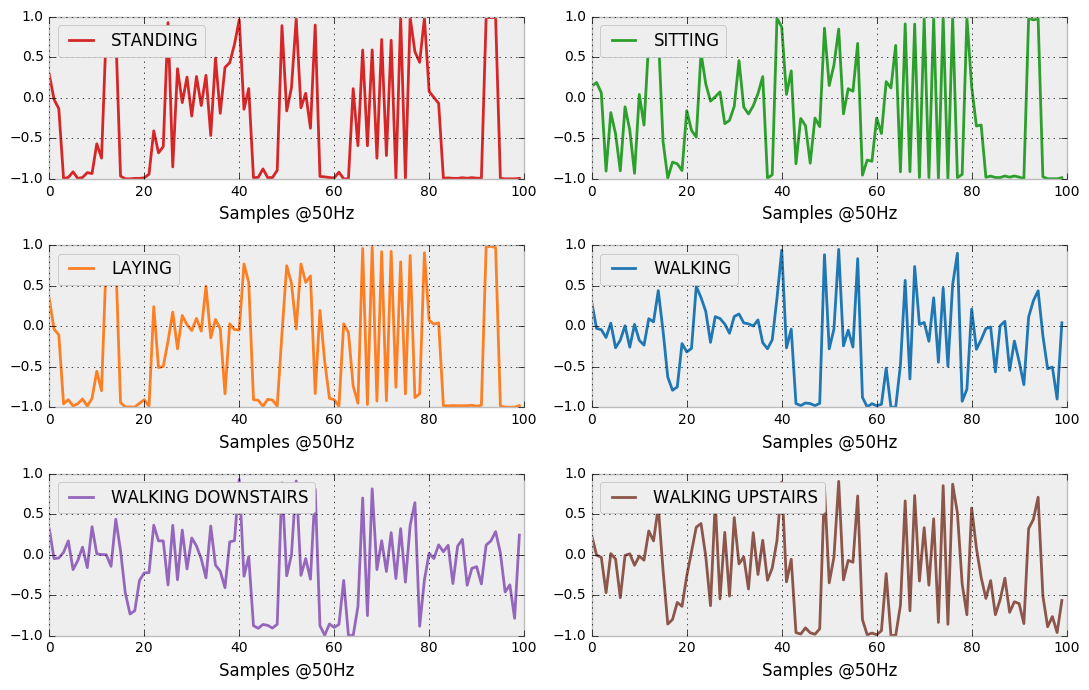#### Model Performance¶

The performance of the KNN & DTW classifier is measured by comparing the class labels from the holdout dataset against predictions made by the classifier. The HAR dataset was pre-split into 70% training and 30% test. Cross validation was not performed.

In :
m = KnnDtw(n_neighbors=1, max_warping_window=10)
m.fit(x_train[::10], y_train[::10])
label, proba = m.predict(x_test[::10])

[****************100%******************]  217120 of 217120 complete

In :
from sklearn.metrics import classification_report, confusion_matrix
print classification_report(label, y_test[::10],
target_names=[l for l in labels.values()])

conf_mat = confusion_matrix(label, y_test[::10])

fig = plt.figure(figsize=(6,6))
width = np.shape(conf_mat)
height = np.shape(conf_mat)

res = plt.imshow(np.array(conf_mat), cmap=plt.cm.summer, interpolation='nearest')
for i, row in enumerate(conf_mat):
for j, c in enumerate(row):
if c>0:
plt.text(j-.2, i+.1, c, fontsize=16)

cb = fig.colorbar(res)
plt.title('Confusion Matrix')
_ = plt.xticks(range(6), [l for l in labels.values()], rotation=90)
_ = plt.yticks(range(6), [l for l in labels.values()])

                    precision    recall  f1-score   support

WALKING       0.96      0.80      0.87        60
WALKING UPSTAIRS       0.85      0.80      0.83        51
WALKING DOWNSTAIRS       0.68      0.97      0.80        31
SITTING       0.78      0.78      0.78        51
STANDING       0.84      0.76      0.80        55
LAYING       0.90      1.00      0.95        47

avg / total       0.85      0.84      0.84       295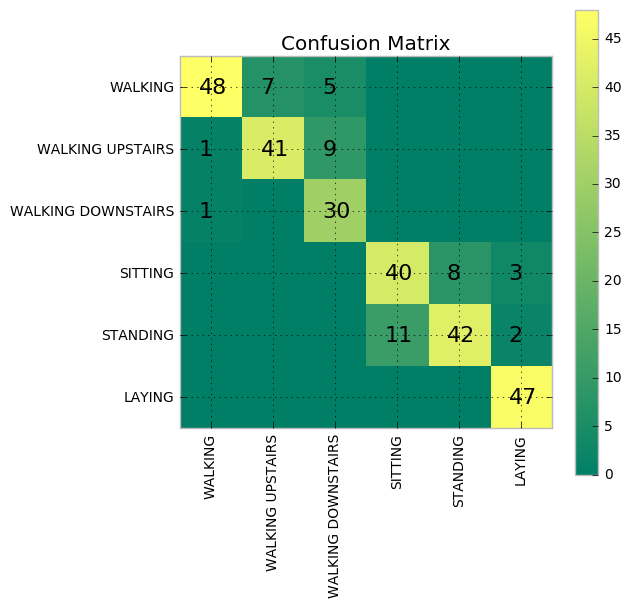The KNN & DTW classifier performed very well across each of the 6 classes. It even beats the best classification rate published by the authors of the HAR paper.

However, this performance comes at a cost. The KNN algorithm is implemented using brute force. So, comparing 500 training observations to 500 test observations results in 250,000 DTW distances to be computed. And each (unconstrained) DTW distance calculation takes over 65K calculations each (256 x 256). This results in 16.3 billion calculations for just 500 x 500 observations.. Clearly not a scalable classification technique!

Performance improvements can be achieved by reducing the max_warping_window parameter. However, these gains will not be sufficient to make KNN & DTW a viable classification technique for large or medium sized datasets.

In :
import time

time_taken = []
windows = [1,2,5,10,50,100,500,1000,5000]

for w in windows:
begin = time.time()

t = KnnDtw(n_neighbors=1, max_warping_window=w)
t.fit(x_train[:20], y_train[:20])
label, proba = t.predict(x_test[:20])

end = time.time()
time_taken.append(end - begin)

fig = plt.figure(figsize=(12,5))
_ = plt.plot(windows, [t/400. for t in time_taken], lw=4)
plt.title('DTW Execution Time with \nvarying Max Warping Window')
plt.ylabel('Execution Time (seconds)')
plt.xlabel('Max Warping Window')
plt.xscale('log')

[****************100%******************]  400 of 400 complete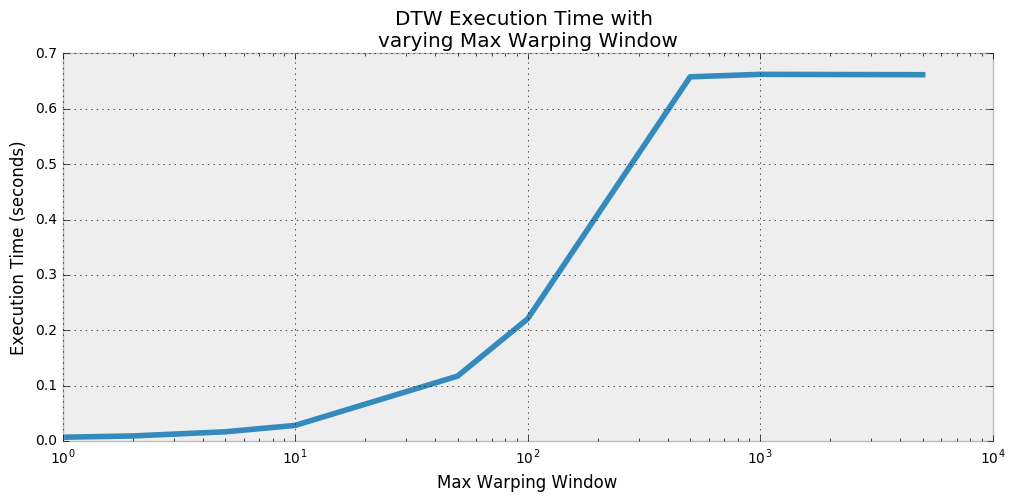#### Final thoughts¶

There are many improvements that could be made to this python implementation of KNN & DTW (eg. rewrite in C++ or explore parallel processing). However, the objective of this project was learning related. I've gained a deeper insight into the underlying mechanics of DTW by implementing it in python using dynamic programming techniques.

1. Mitsa (2010). Temporal Data Mining (Chapter on Temporal Classification).
2. Xi (2006). Fast Time Series Classification Using Numerosity Reduction.
3. Davide Anguita, Alessandro Ghio, Luca Oneto, Xavier Parra and Jorge L. Reyes-Ortiz. Human Activity Recognition on Smartphones using a Multiclass Hardware-Friendly Support Vector Machine. International Workshop of Ambient Assisted Living (IWAAL 2012). Vitoria-Gasteiz, Spain. Dec 2012. Read Paper

#### Credit¶

• The progressbar used in the DtwKnn() class was taken from PYMC
• The matplotlib style and IPython notebook was taken from Cameron Davidson-Pilon's excelent "Bayesian Methods for Hackers"
In :
from IPython.core.display import HTML

def css_styling():